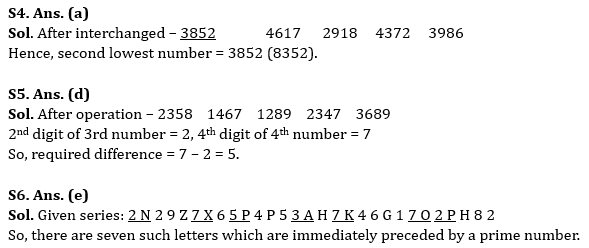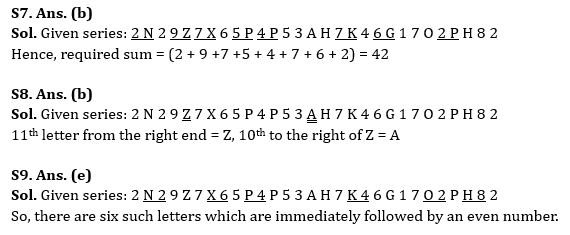Latest Banking jobs   »

# Reasoning Quizzes Quiz For IBPS RRB PO/Clerk Prelims 2023-22nd May

Directions (1-5): Answer the questions based on the information given below.

8352 6417 9218 3472 9386

Note: The 1st, 2nd, 3rd and so on numbers or digits or elements are taken from left end.

Q1. If 1 is added to 2nd and 3rd digits of all the given numbers, then how many numbers will have more than two even digits?
(a) One
(b) Two
(c) Three
(d) More than three
(e) None of these

Q2. If digits of all the given numbers are arranged in decreasing order within the number, then what will be the sum of 3rd digit of 2nd number and 2nd digit of 5th number?
(a) 11
(b) 13
(c) 12
(d) 15
(e) None of these

Q3. If digits of all the numbers are added, then which number obtains the lower sum?
(a) 8352
(b) 3472
(c) 9218
(d) 9386
(e) None of these

Q4. If 1st and 2nd digit of each number is interchanged, then which number will be the second lowest?
(a) 8352
(b) 3472
(c) 9218
(d) 9386
(e) 6417

Q5. If digits of all the given numbers are arranged in ascending order, then what will be the difference of 2nd digit of 3rd number and 4th digit of 4th number?
(a) 10
(b) 6
(c) 8
(d) 5
(e) None of these

Direction (6-10): Study the following series carefully and answer the questions accordingly.

2 N 2 9 Z 7 X 6 5 P 4 P 5 3 A H 7 K 4 6 G 1 7 O 2 P H 8 2

Q6. How many letters are immediately preceded by a prime number?
(a) One
(b) Two
(c) Three
(d) Four
(e) None of the above

Q7. What will be the sum of all digits which are immediately succeeded by a consonant?
(a) 40
(b) 42
(c) 44
(d) 46
(e) None of the above

Q8. Which element is 10th to the right of 11th letter from right end?
(a) Z
(b) A
(c) X
(d) 6
(e) None of the above

Q9. How many letters are immediately followed by an even number?
(a) One
(b) Two
(c) Three
(d) Four
(e) More than four

Q10. How many letters are in between 4th number from left end and 5th number from right end?
(a) Five
(b) Six
(c) Seven
(d) Eight
(e) None of the above

Solutions

Solution (1-5):
S1. Ans. (a)
Sol. After operation – 8462 6527 9328 3582 9496
Hence, there is one number (8462) which has more than two even digits.

S2. Ans. (c)
Sol. Decreasing order – 8532 7641 9821 7432 9863
3rd digit of 2nd number = 4, 2nd digit of 5th number = 8, So, required sum = 4 + 8 = 12

S3. Ans. (b)
Sol. After operation – 18 18 20 16 26
Hence, 3472 obtains the lower sum.S10. Ans. (c)
Sol. Given series: 2 N 2 9 Z 7 X 6 5 P 4 P 5 3 A H 7 K 4 6 G 1 7 O 2 P H 8 2
4th number from the left end = 7, 5th number from the right end = 1
Hence, there are seven letters between them.## FAQs

### How many sections are there in the IRDAI exam?

There are 4 sections in the IRDAI assistant manager online preliminary exam. They are Reasoning, English Language, General awareness, Quantitative aptitude.

#### Congratulations!Union Budget 2023-24: Free PDF